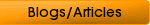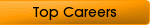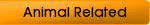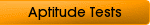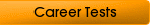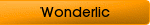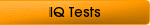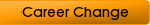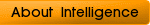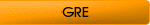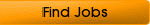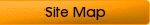## GMAT Problem Solving Strategies for taking the Test

GMAT problem solving questions are about determining your understanding of mathematical concepts rather than how you arrive at the answer. But, your problem solving skills may be your weak point, and you need to pass the GMAT for acceptance into business school.

You have nothing to worry about if you have a basic understanding of mathematical principles. You are not tested on advanced mathematical principles such as calculus and trigonometry.

All you need to do is a little preparation and you should get the scores you need for acceptance into the school of your choice. You should have spent the last few months preparing for taking your GMAT, so you should be ready to sit down and take your test.

It is important to read each question carefully. One of the pitfalls of the GMAT is that people answer what they think they read, instead of the question the test actually asks. The questions are deliberately written to trip you up. For example, look at the difference between “Which of the following are true?” and “Which of the following are not true?”

There is nothing worse than getting the results of your test back and seeing you misunderstood the questions.

### Use Scrap Paper to Work out the Answers

Do not forget to take a pad of scrap paper into the GMAT with you because you need it to help you answer the problem solving section of the GMAT. Using the paper helps you to avoid mistakes and the traps in the multiple choice answers.Once you enter your answer onto the test you cannot change, so you want to be sure the first time.

### Do not Waste Time

Do not spend much time trying to calculate the answers as the questions are designed to need the minimum amount of calculation to reach a correct answer. If you find yourself spending too much time working out an answer, then there is something you are overlooking.

### Use the Guesstimating Technique

Normally the answer to GMAT problem solving questions requires a numerical value from multiple choice answers. Usually the choices in the answers are not close to each so you can roughly guesstimate the correct answer. For example, if you roughly know the answer to the question is 25 percent, and your choice of answers is 13 percent, 26 percent, 32 percent, and 41 percent then you can safely guess that 26 percent is the correct answer.Using this technique will save you a lot of time and long, convoluted calculations that often open you up to making a mistake.

### How to Work Backwards

If you are finding it difficult to answer a question try choosing an answer and work backwards to see if it fits in. When you use this technique, start at the middle answer and, even if it is not the right answer, it will help you to find out if the answer is higher or lower. You are one step closer to the correct answer.

### Geometry Problem Techniques

Always assume that any pictures and graphics are accurate unless specifically told otherwise. One of the most common mistakes made when answering GMAT problem solving questions is relying solely on your visual judgment. Make sure you read the text in line with the specific graphic before choosing an answer.

The illustrations and diagrams have many hidden numbers and facts; make sure you spend at least 30 seconds reviewing each drawing before choosing your answer. You may need to do a quick sketch of the drawings to work out the facts hidden in the data.

### Graph Solving Techniques

Make sure you spend some time reviewing tables and graphs to understand the information contained within them. Graph problems do not require any sort of calculation. Instead, they determine your ability to interpret the data. Remember, the units of measurement, axis labels, and the legend key will give you many valuable keys to the answer.

### Word Problem Solving Techniques

One section of the mathematical problem solving is a series of mathematical problems presented in word format. For example, if the red car is traveling at a certain speed how many miles will it travel in how many hours?

To help you choose the right answer, build an equation on your scrap paper using symbols such A for speed, B for miles, and C for hours. These can help you to make calculations.

Home
GMAT Sample Test
GMAT PracticeTests
GMAT Tips
GMAT Scores
GMAT Sample Questions